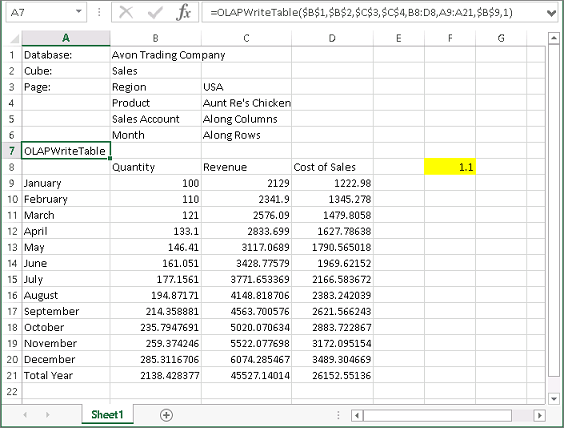OLAPWriteTableThe OLAPWriteTable is the enhanced version of the OLAPTable function in that it can function as a regular OLAPTable but it can also be used to create a formula in Excel which will actually recalculate or change the particular data point in PowerOLAP® but it will still retain the formula in the corresponding cell in Excel. The OLAPWriteTable consists of four modes: (1) Display Cube Values, (2) Use Excel Formulas, (3) Commit formula values to Cube, and (4) Clear formulas and display cube values.

To access the different OLAPWriteTable modes, simply double click on the cell containing the OLAPWriteTable function. This action will bring up a dialog box which drops down to the different modes available and users can easily select the desired option/mode to be used. By default, the OLAPWriteTable function is set to Display Cube Values mode.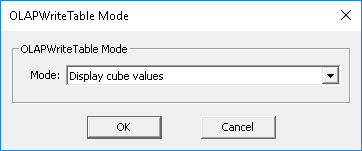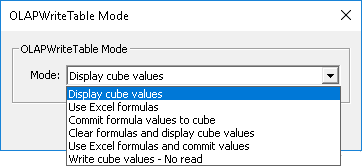The advantage of using this function is that it enables a user to work in multiple modes. On top of being able to enjoy the functionalities of working with an Excel worksheet that is dynamically linked to a database, a user also is provided with an alternative option for populating a corresponding database through the use of Excel formula driven values, instead of having to define them from within the PowerOLAP application.

This function will be extremely beneficial to those users who are not familiar or who do not have an adequate knowledge and understanding of the cube formula creation in a PowerOLAP database. By setting the OLAPWriteTable to be in the ‘Use Excel Formulas Mode‘ (2nd Mode), any user can perform simple formula calculation directly within Excel and push the result back to the PowerOLAP database.

One of the parameters of this function is it needs to be flagged as either “1” ,”2″, “3” or “4” which will dictate or determine the mode that will be used by the OLAPWriteTable function.

Mode 1: Display Cube Values

By specifying “1” in the parameter, it actually sets OLAPWriteTable function to be in the ‘Display Cube Values’ mode, which pretty much resembles the OLAPTable function. Using this mode, PowerOLAP will send and update data in your Excel worksheet in a table form. Likewise, it also has a writeback capability, wherein you can change the numbers or fact data within the range and those values will be pushed back to the corresponding database. The OLAPWriteTable mode is set to use this mode by default.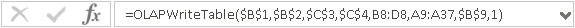OLAPWriteTable: In Display Cube Values Mode

Mode 2: Use Excel formulas

By specifying “2” in the parameter, it sets OLAPWriteTable function to be in the ‘Excel Formula’ mode, which can be used when you want to change a data point in PowerOLAP using formulas written in Excel. When the OLAPWriteTable function is set to this mode, users can create formulas within the Excel worksheet which is dynamically connected to a PowerOLAP database, and commit the results of those formulas back to the corresponding database. Even after resfreshing the worksheet, the corresponding cells containing the formulas will still be able to retain the formulas used. Notice that cells containing excel formula driven values are marked with cell/comment indicators.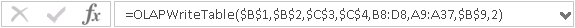OLAPWriteTable: Excel Formula Mode

Mode 3: Commit formula Values to Cube

By specifying “3” in the parameter, it sets the OLAPWriteTable function to be in the ‘Commit formula values to cube’. This is used when you wish to commit the results of formula calculations in excel to the corresponding database. This mode is particularly useful when you want to write a formula or similar formula that will apply to several cells or data points of a PowerOLAP database. For example, from within a fact data array on your worksheet, you can write a formula in a particular cell using the ‘Excel Formula ‘ mode. However, since you know that the same formula needs to be applied to several other cells, instead of writing the formula for each of those corresponding cells, you can simply copy then paste the formula in the appropriate cells. After refreshing or recalculating the values in the Excel worksheet, switch to the ‘Commit formula Values to Cube’ mode to update the database of the calculation results.

Notice that after the figures have been written back to the database, the cell containing the OLAPWriteTable function automatically switches back to ‘Excel Formula’ mode.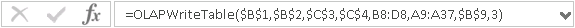OLAPWriteTable: Commit Formula Values to Cube Mode

Mode 4: Clear formulas and display cube values

By specifying “4” in the parameter, it sets the OLAPWriteTable function to be in the ‘Clear formulas and display cube values’ mode. Switching to this mode will rip off all the formulas in the slice to Excel report but will retain the calculated result/data in the cells, as well as keep those calculated data in the corresponding database.

Notice that the comment indicators are removed on the cells, and the formulas are removed. Additionally, clicking on the cell containing the OLAPWriteTable function shows that it automatically switched back to the ‘Display Cube Values’ mode.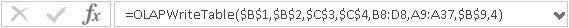OLAPWriteTable: Clear Formulas and Display Cube Values Mode

Mode 5: Use Excel fomulas and commit values

By specifying “5” in the parameter, it sets the OLAPWriteTable function to be in the ‘Use Excel formulas and commit values’ mode. This option allows you to write an Excel formula in an OLAPTable then have those formulas apply directly to the PowerOLAP cube. For example, say you want a cell in your OLAPTable, C1 to be the sum of an Excel formula A1 + B1. Every time you enter a new value in A1 or B1 and recalculate, you want C1 to write back its new value to PowerOLAP. You would not be able to do this with a regular OLAPTable. This feature allows users to stay in Excel and not have to do stuff in the modeler.

To demonstrate OLAPWriteTable functionality and how the different modes works

1.   Open a slice in the appropriate database.
In the following example, a slice is created out of the Sales cube from the PowerOLAP sample database called Avon Trading Company.

2.   From the PowerOLAP ribbon go to the Slice tab, then in the Worksheet control group, click on the Worksheet drop down command button and select OLAPWriteTable option.

Alternatively, you may go to Home tab, select Options command button, then go to the General tab and pick OLAPWriteTable as the Excel function. Next, with the slice opened in the application, select the Slice tab from the PowerOLAP ribbon and click on the Worksheet button.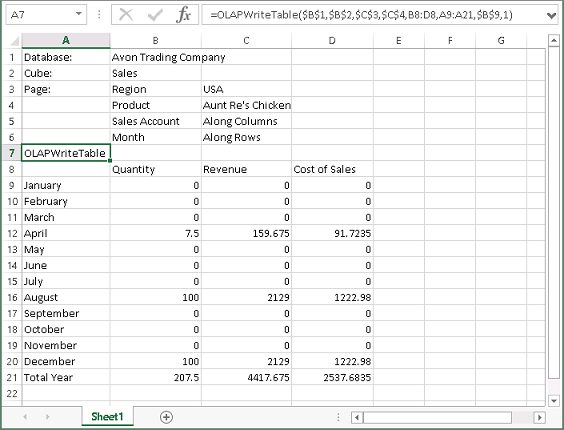Using OLAPWriteTable: Display Cube value Mode (1)

Clicking on the OLAPWriteTable functions shows that the last parameter is set to 1, which indicates that the Excel function is set to ‘Display Cube values’ mode.

3.   Click on the cell corresponding to Quantity for the month of January (cell B9 in the screenshot) and change the value to 100.

When you refresh the value, then go back to the corresponding database in PowerOLAP and recalculate, you will see the corresponding intersection gets updated as well.

Next, we will try to create a formula in the Excel report and use that formula to derive the values that we want to put in PowerOLAP.

Using OLAPWriteTable: Use Excel Formulas Mode (2)

4.   Double click on the OLAPWriteTable function and pick ‘Use Excel Formulas‘ option from the dialog that appears.

Next, assuming that you want the Sales quantity for each month to be 10 percent more than that of the prior month.

5.   Go to cell F8 and type 1.1 (equivalent to 110 %).

6.   In the cell corresponding to sales Quantity for February (cell B10 in the screenshot), type the formula equal to Sales Quantity for January * 1.1 (make sure that in the formula, the cell corresponding to 1.1 should be formatted to be an absolute reference).

7.   Press F9 or recalculate the worksheet.

Notice in the worksheet that after recalculation, the cell corresponding to Quantity for February appears marked with a cell/comment indicator. Even after refreshing the values or clicking from another cell and back will retain the formula that is used to derived the value returned in the cell.

Going to the corresponding database and recalculating it will show the data is updated in the corresponding intersection.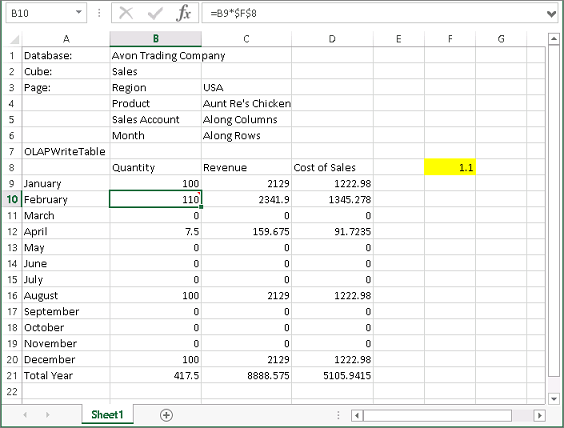Using OLAPWriteTable: Commit formula Values to Cube Mode (3)

Next, assuming that you want to apply the same formula, wherein March quantity will also be 10% more than February and so on (For this example, round of the result to a whole number).

8.   Copy and paste the formula from cell B10 to the cells corresponding to Quantity for March until December (in the example, paste to cell B11 to B20).

9.   Press F9 or recalculate the worksheet.

Notice that the rest of the cells are now also marked with cell/comment indicator, and that the Quantity for each month is indeed 110% percent of the prior month.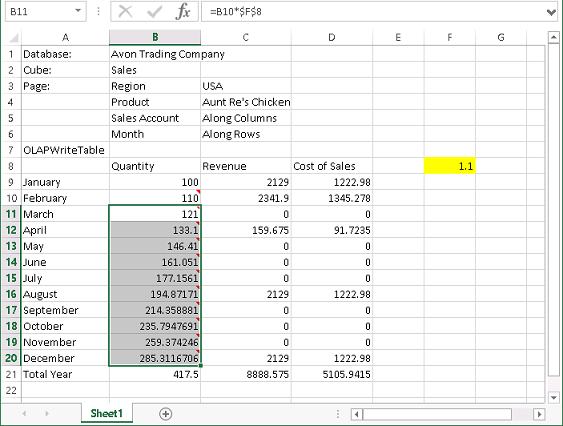However, if you check the corresponding database and recalculate, the data for March until December does not appear to be written back into the database.

10. Double click on the OLAPWriteTable function and pick ‘Commit formula Values to Cube‘ option from the dialog that appears.

Now, if you check the PowerOLAP database, you will see the numbers did come in.

If you look into the Excel worksheet, notice that the affected cells still retained the formulas that was used to calculate for the values. Additionally, if you check the cell containing the OLAPWriteTable function, you will see that the mode automatically switched back to the ‘Excel Formula’ mode or the second mode.

Using OLAPWriteTable: Clear formulas and display cube values (4)

For example, the desired resulting values are already achieved, and you prefer to remove all the formulas that were written in Excel, but still retain the calculated values in your worksheet.

11. Double click on the OLAPWriteTable function and pick ‘Clear formulas and display cube values‘ option from the dialog that appears.

12. Press F9 or recalculate the worksheet

Notice that the cell/comment indicators disappeared. If you now click on the individual cells wherein formulas were previously applied, you will see that those formulas no longer exists, and all that was retained were the calculated figures or numbers.

Additionally, clicking on the cell containing the OLAPWriteTable now shows that it automatically switched to the first mode or the ‘Display Cube Values’ mode.